## short communicationsFOUNDATIONSADVANCES
ISSN: 2053-2733
Volume 77| Part 4| July 2021| Pages 257-261

## Multiwavelength anomalous X-ray diffraction for combined imaging of atomic displacement and strain

aDepartment of Physics, University of California San Diego, La Jolla, CA 92093, USA
*Correspondence e-mail: a.shabalin.r@gmail.com, oshpyrko@ucsd.edu

(Received 4 December 2020; accepted 11 May 2021; online 21 June 2021)

The X-ray Bragg coherent diffractive imaging (CDI) technique assumes that the structure factor holds constant over the measured crystal. This approximation breaks down for materials exhibiting variations in the unit-cell configuration, such as piezo- and ferroelectrics. In that case, the strain field cannot be reliably determined from the reconstruction because the lattice deformation and the structure factor contribute concomitantly. Proposed here is a solution to this problem achieved by combining Bragg CDI and the multiwavelength anomalous diffraction approach that measures a Friedel pair of reflections at two different photon energies near an absorption edge. Comparing the obtained reconstructions with a parametric model that includes calculating the scattering amplitude as a function of wavelength and the unit-cell configuration, the contributions of the lattice deformation and the structure factor are separated. Simulations of the ferroelectric material BaTiO3 demonstrate the possibility of simultaneous probing of the strain and displacement of the Ti atoms. The proposed method opens up an opportunity to apply coherent X-ray diffraction for nanoscale-resolved 3D mapping of polarization domains in micro- and nanocrystals.

### 1. Introduction

Strong coupling between lattice strain and polarization is one of the most useful features of piezo- and ferroelectrics (Bian et al., 2019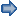; Alsubaie et al., 2018). The functional properties of these materials originate in the local energy landscape and the resulting domain structure, typically on the mesoscale (Tagantsev et al., 2010). Therefore, understanding the mechanisms underpinning the interplay of strain and polarization domains is important for both fundamental and techno­logical perspectives. This motivates the development of experimental techniques for simultaneous 3D mapping of both polarization and strain in micro- and nanocrystals.

Addressing this challenge requires using a highly penetrating and non-destructive probe sensitive to both the crystal lattice deformations and the unit-cell distortions. In this regard, X-ray Bragg coherent diffractive imaging (CDI) has been established as a remarkably successful in situ method that allows probing of the 3D strain landscape in individual crystals with 10–100 nm resolution (Pfeifer et al., 2006; Ulvestad et al., 2015). However, applying this technique to inhomogeneously polarized materials remains challenging due to the structure factor's intrinsic dependence on the atomic displacements that underlie the emergent polarization state (Lines & Glass, 2001). Decoupling the lattice deformation field and the polarization field in the reconstructed 3D phase distribution requires twice as much information as that contained in a single Bragg CDI scan because the number of unknown variables is doubled. Here we suggest a method for deriving those 3D distributions from two datasets measured at different photon energies near an X-ray absorption edge for one of the constituent atoms.

Our approach takes advantage of the sensitivity of the structure factor to the dispersion corrections in the resonant atom's scattering contribution (Favre-Nicolin et al., 2012). Such an effect can be further facilitated by extracting the difference between the complex scattered amplitudes for reflections with reciprocal-lattice vectors h and(a Friedel pair). This concept is actively used in the multiwavelength anomalous diffraction technique (MAD) (Hendrickson, 1991) and has recently been applied to the structure–property characterization of ferroelectrics (Gorfman et al., 2016). Here we propose that combining the MAD approach with Bragg CDI would enable mapping of the atomic displacement in addition to the strain. Considering a model case of the ferroelectric material BaTiO3, we demonstrate the possibility of retrieving the 3D distributions of the lattice deformation and the unit-cell displacement of the Ti atom.

The application of X-ray microscopy for imaging polarization in ferroelectrics has already been advanced by the following reports. Do et al. (2004) used the variations in Bragg peak intensity in microdiffraction for 2D mapping of domains in a thin PZT film. Hruszkewycz et al. (2013) obtained the out-of-plane polarization component in a thin PbTiO3 film from the reconstructed phase in 2D Bragg ptychography. Finally, Karpov et al. (2017) used the combination of phase-field simulations with Bragg CDI measurements to probe indirectly the local polarization state in a BaTiO3 nanoparticle. We hope that our work will add another step towards achieving high-resolution 3D imaging of polarization and strain.

### 2. Theory

In a typical Bragg CDI experiment, a finite crystalline sample is illuminated by an intense coherent X-ray beam and oriented to fulfill a Bragg diffraction condition. The resulting far-field interference pattern around the chosen Bragg peak is recorded with a 2D detector [see Fig. 1(a)]. Different cross sections through reciprocal space are accessed by rocking the sample in the beam so that a full 3D dataset usually consists of a few tens of diffraction patterns.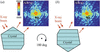Figure 1 Schematic diagrams of the multiwavelength anomalous Bragg CDI approach. (a) First, Bragg CDI datasets for the h reflection are collected at two photon energies: on the absorption edge of the anomalously scattering atom and several electronvolts below it. (b) Second, the sample is rotated by 180°, and then both measurements are repeated, thus providing two Bragg CDI datasets for thereflection.

Under the approximation of kinematic diffraction, the scattered amplitude in the vicinity of a reflection with the reciprocal lattice vector h is given by a Fourier integral (Shabalin et al., 2017),Here h + q is the momentum transfer vector, P(r) is the probe function and Fh(r) is the structure factor. The object function is expressed asin which sh(r) denotes the crystal density function and u(r) is the lattice deformation field.

Equation (1) serves as the foundation of the Bragg CDI method because it establishes the connection between the measured amplitudes in reciprocal space and the object function in real space (Vartanyants & Yefanov, 2015). The phase of A(h + q) is not measured directly but recovered using iterative phase retrieval algorithms (Fienup, 1982). The resulting reconstruction is determined up to some complex constant and interpreted as proportional to the object function provided by equation (2).

In conventional Bragg CDI experiments, it is assumed that Fh(r) does not vary across the crystal, which allows Fh(r) = Fh to be taken out of the integral. This approximation holds well for many experimental cases, but there are exceptions in which the atomic arrangement in the unit cell varies over the crystal volume. For example, in piezo- and ferroelectrics, the polarization typically originates from asymmetry in the unit cell's charge distribution (Konishi et al., 2016). The displacement of ions from their average position changes between different domains (Jia et al., 2007), and so do the amplitude and phase of the structure factor. As a result, the reconstruction cannot be directly interpreted as the object function sh(r) exp[−ih·u(r)] because Fh(r) cannot be taken out of the integral.

In these abnormal cases, the structure factor in equation (1) is a non-uniform 3D distribution, which doubles the number of unknown variables in the model. Therefore, separating the contributions of the structure factor Fh(r) and the deformation field u(r) requires an additional measurement in which these functions would contribute differently. We propose achieving this by varying the X-ray photon energy across one of the absorption edges. The dispersion corrections make the structure factor sensitive to such variations, while the contribution from lattice deformation remains constant.

Let us consider a unit cell in which one of the atomic positions (pa) varies across the crystal, while all other atomic positions are fixed. Taking into account the dispersion corrections, we express the structure factors for reflections with the reciprocal-lattice vectors h andaswhere f0 + f′ + if′′ is the scattering factor of the shifting atom, andis the sum of scattering contributions from the fixed atoms. The angle betweenand f0 [see Fig. 2(a)] is composed of its average value (ψc) and an additional phase h · Δpa(r) introduced by the local deviation of pa(r) from its average value. As we show in Appendix A, this angle can be expressed asEquation (4) can be used for mapping Δpa(r), that is the local displacement of the anomalously scattering atom in the unit cell. For that, one has to calculatetheoretically, measureand |Fh(r)|2, and then estimate ψc using the average values of those structure factors.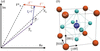Figure 2 Structure factor of polarized BaTiO3. (a) Argand diagram for the Friedel pair of reflections. The sum of contributions from non-resonant atoms is denoted. The anomalously scattering atom contributes with an atomic scattering factor, including the dispersion corrections. The relative phase between the contribution from this atom and all the other atoms is denoted ψ. (b) The tetragonal unit cell of polarized BaTiO3. The arrow illustrates that polarization emerges due to the displacement of the Ti4+ ion along the  direction.

In the Bragg CDI reconstruction, the structure factor contribution is multiplied by the contributions of the lattice displacement and the probe function [see equations (1) and (2)]. To eliminate these two contributions not depending on the photon energy, we compute the ratio of reconstructions(See the detailed derivation in Appendix B). Here, Rh, res and Rh, off are the reconstructions for reflection h measured on and off the resonant energy, respectively. Similarly,andare the reconstructions for reflectionmeasured at the same photon energies. In equation (5) the normalization of one reconstruction by another helps to eliminate errors arising due to possible changes in the beam intensity. After ξ(r) is obtained, the structure factor and the resonant atom position pa(r) can be computed. In the next step, this allows the separation of the structure factor contribution from the reconstruction and the attribution of the residual phase to the lattice deformation.

### 3. Simulations

We performed simulations of the ferroelectric material BaTiO3 [space group P4mm, Inorganic Crystal Structure Database (ICSD; http://icsd.fiz-karlsruhe.de/icsd/) code 245944], in which the polarization emerges mainly due to the off-center displacement of the Ti4+ ion along the  crystallographic direction (Zhang et al., 2006) [see Fig. 2(b)]. Figs. 3(a) and 3(b) show the calculated structure factors for the 002 andreflections at two X-ray photon energies: the Ti K absorption edge (4.967 keV) and 2 eV below that. The results demonstrate the high sensitivity of the amplitude and phase of the structure factor to the unit-cell configuration and the dispersion corrections. This makes it possible to determine the magnitude of the Ti displacement by comparing the experimentally obtained value of the parameter ξ with the theoretical one. This concept is illustrated in Fig. 4, where we plot the real and imaginary parts of the parameter ξ and mark data points for a few values of the Ti atom displacement.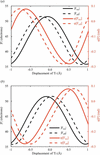Figure 3 Calculations of the structure factor for the () reflections of BaTiO3 as a function of the Ti atom displacement along the  crystallographic direction. Amplitudes (black curves) and phases (red curves) of structure factors are plotted for (a) the 002 and (b) thereflections of BaTiO3. Solid curves (res') represent calculations for X-ray photon energy equal to the Ti K absorption edge (4.967 keV). Dashed curves (off') represent calculations for X-ray photon energy 2 eV below that.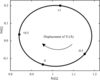Figure 4 Parameter ξ calculated for the () reflections of BaTiO3, the Ti K absorption edge, and the Ti atom displacements in the range of [−1; 1] Å. Real (x axis) and imaginary (y axis) parts of parameter ξ are plotted. The arrow and labels mark Ti atom displacement along the  direction in the tetragonal unit cell of BaTiO3.

The case of a non-distorted unit cell is marked as `0', and for positive values of displacement the data point is shifted in the clockwise direction. Such a trajectory reflects the geometry in which the Ti atomic scattering factor contributes to the structure factor, as shown schematically in Fig. 2(a). Displace­ment of the Ti atom modifies the phase at which its scattering contribution is added so that 2π (the full loop) corresponds to the period of 1/|h| = dhkl. For the considered crystal structure, this period is 2 Å; therefore, the displacement range from −1 to 1 Å covers the full loop. Finally, we suggest that fitting the absolute phase of the experimentally obtained distribution of ξ(r) to match the computed one can help in minimizing possible errors introduced by the phase retrieval algorithm.

### 4. Discussion and conclusion

The following constraints may limit the potential of our method. First, since the structure factor is sensitive only to the atomic displacements parallel to the scattering vector, probing the other components of polarization and strain would require measuring more than one pair of reflections. Moreover, as the method relies on modeling it might fail to deal with complicated structures such as those in which using a single fitting parameter is not sufficient to characterize all the variations in the unit-cell configuration. Second, artifacts due to imperfections in the experimental conditions and the phase retrieval algorithms might obscure structure factor variations. Dealing with such artifacts could be problematic if the dispersion corrections are weak compared with all the other scattering contributions. Third, since the photon energy has to coincide with one of the absorption edges, the Q range and, consequently, the range of measurable reflections are limited. Generally, high-order reflections benefit from enhanced sensitivity of the structure factor to the atomic displacements but suffer from lower dispersion corrections (because using a high photon energy is required). We emphasize that our method is not limited to the absorption edge of specifically the shifting atom – in principle, an absorption edge of any atom in the unit cell can be used.

In conclusion, we propose a method for decoupling the contributions of the structure factor and the lattice deformation in Bragg CDI experiments. Similar to the MAD approach, it relies on measuring two reflections belonging to a Friedel pair. For each reflection, a Bragg CDI dataset is acquired using two photon energies: at the absorption edge for one of the atoms constituting the crystal unit cell and several electronvolts below that edge. Simulations performed for the ferroelectric material BaTiO3 demonstrate the possibility of mapping both the lattice deformation and the Ti atom's off-center displacement. Our method paves the way to using coherent X-ray scattering for 3D nanoscale imaging of polarization domains in piezo- and ferroelectrics.

### Derivation of equation (4)

Taking into account the dispersion corrections for the anomalously scattering atom, the structure factor's modulus Fh can be expressed as[See also the diagram in Fig. 2(a)]. Similarly, for the opposite reflection we writeConsequently, the difference between these modules is expressed as### Derivation of equation (5)

First, we write down the ratio of reconstructions for the h reflection,Second, we write down the ratio of reconstructions for thereflection,Dividing equation (10) by equation (11) and neglecting the off-resonant factor(due to the smallness of), we straightforwardly obtain equation (5).

### Funding information

This research was supported by US Department of Energy, Office of Science, Office of Basic Energy Sciences, under contract No. DE-SC0001805.

### ReferencesFOUNDATIONSADVANCES